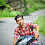# 10th Class NCERT (CBSE) Mathematics | Chapter 1, REAL NUMBERS | Math Textbook Exercise 1.4 (Solution)

Class 10, Ncert Cbse Mathematics
Chapter 1 Real Numbers
NCERT Math Solutions (Exercise 1.4)
(Page 17)
Q1: Without actually performing the long division, state whether the following rational numbers will have a terminating decimal expansion or a non-terminating repeating decimal expansion:

Solution:

The denominator can be written in the form 5m. Hence, this decimal expansion is terminating.

Since the denominator is of the form 2m so, this decimal expansion is terminating.

Since the denominator can not be written in the form 2m × 5n and it also contains other factors 7 and 13, so its decimal expansion will be non-terminating repeating type.

As the denominator is of the form 2m × 5n so, the above decimal expansion is terminating.

Since the denominator can not be written in the form 2m × 5n and it has 7 as its factor so, the decimal expansion of is non-terminating repeating.

The denominator is of the form 2m × 5n therefore, it is a terminating decimal expansion.

Since the denominator is not of the form 2m × 5n, and it also contain another factor 7 so, the above decimal expansion is non-terminating repeating.

The denominator can be written in the form 2m × 5n. Hence, the above decimal expansion is terminating.

Since the denominator is of the form 2m × 5n so, the above decimal expansion is a terminating one.

Since the denominator is not of the form 2m × 5n and also contains 3 as its factors so, the above decimal expansion is non-terminating repeating.
Q2: Write down the decimal expansions of those rational numbers in Question 1 above which have terminating decimal expansions.
Solution:

Q3: The following real numbers have decimal expansions as given below. In each case, decide whether they are rational or not. If they are rational, and of the form p/q, what can you say about the prime factor of q?

Solution:
(i) 43.123456789
This number has a terminating decimal expansion. So, it is a rational number of the form p/q and q is of the form
(ii) 0.120120012000120000 …
The given decimal expansion is non-terminating and non-recurring. Therefore, it is an irrational number.

Since the given decimal expansion is non-terminating, so it is a rational number of the form p/q and q is not of the form 2m x 5n i.e., the prime factors of q will also have a factor other than 2 or 5.

1.2.